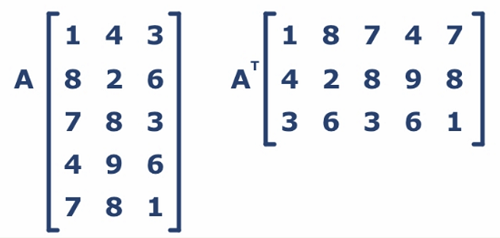# Transpose Matrix | Linear Algebra using Python

Linear Algebra using Python | Transpose Matrix: Here, we are going to learn how to print the transpose matrix in Python?
Submitted by Anuj Singh, on May 26, 2020

Prerequisites:

The transpose of a matrix is a matrix whose rows are the columns of the original. In mathematical terms, A(i,j) becomes A(j,i) in the new matrix. Transpose has an important role in understanding and implementing Machine Learning algorithms. Major usage by Matrix Multiplication.Method 1:

```Syntax:
transpose_M = M.T
Parameter:
Matrix M
Return:
MT
```

Method 2:

```Syntax:
transpose_M = numpy.transpose(M)
Input Parameter:
Matrix M
Return:
MT
```

## Python code for transpose matrix

```# Linear Algebra Learning Sequence
# Transpose using different Method

import numpy as np

g = np.array([[2,3,4], [45,45,45]])
print("---Matrix g----\n", g)

# Transposing the Matrix g
print('\n\nTranspose as g.T----\n', g.T)
print('\n\nTranspose as np.tanspose(g)----\n', np.transpose(g))
```

Output:

```---Matrix g----
[[ 2  3  4]
[45 45 45]]

Transpose as g.T----
[[ 2 45]
[ 3 45]
[ 4 45]]

Transpose as np.tanspose(g)----
[[ 2 45]
[ 3 45]
[ 4 45]]
```

Preparation

What's New

Top Interview Coding Problems/Challenges!